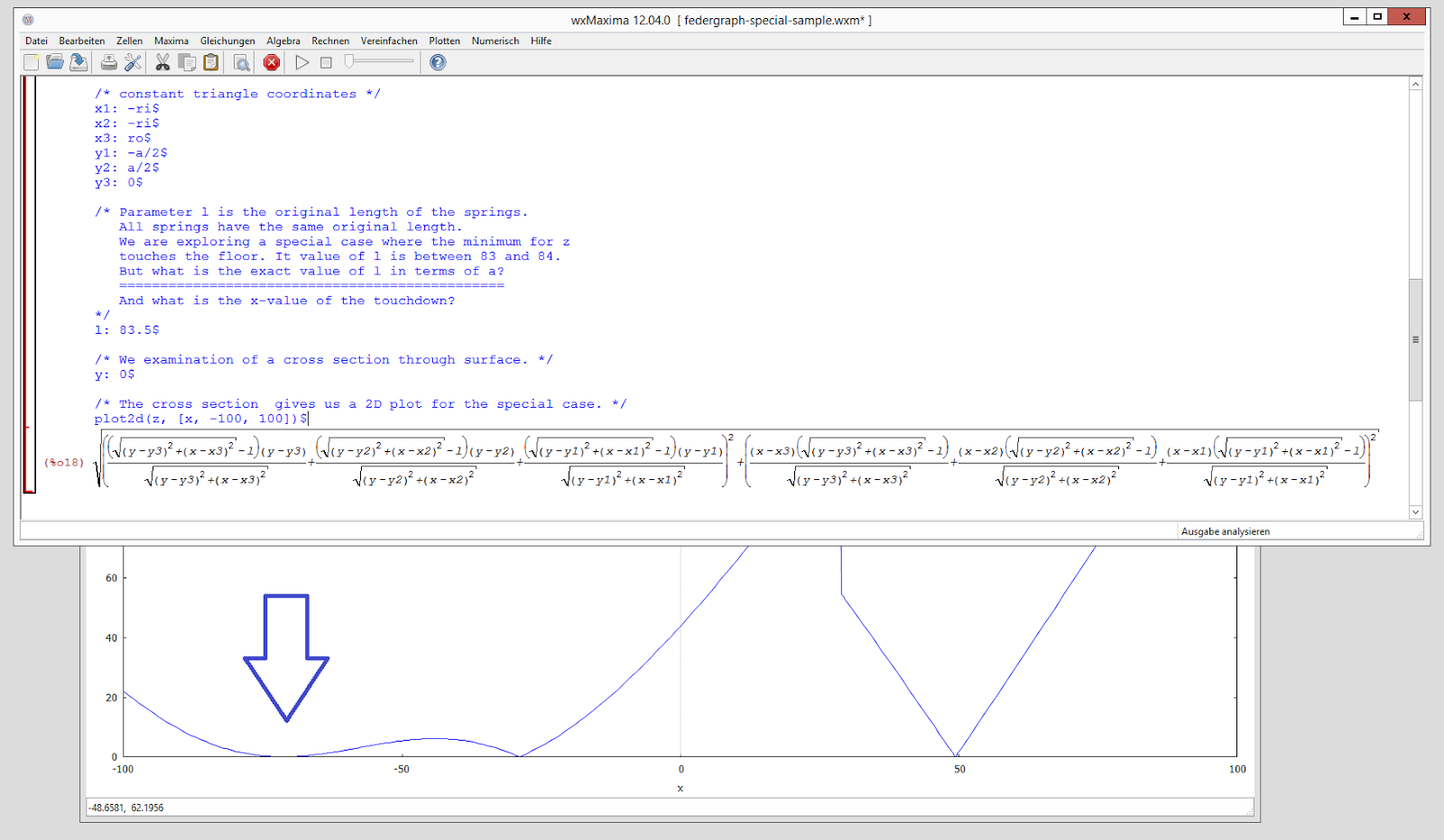# A Challenge

I know you won’t take it.

But for the record, I put this up, back then, at the date of the blog post, and now (in 2019) I have just recycled the post, it reads as follows:

“I have updated the Maxima source for the special sample, made a screenshot, and wrote a draft for a challenge/task in notepad. Perhaps I will post it to the forum. This is certainly not the biggest or most urgent problem to solve, but perhaps also not the smallest or least interesting.”See source code below.

Maxima source code:

``````kill(all)\$

a1: (x-x1)^2 + (y-y1)^2\$
a2: (x-x2)^2 + (y-y2)^2\$
a3: (x-x3)^2 + (y-y3)^2\$

t1: sqrt(a1)\$
t2: sqrt(a2)\$
t3: sqrt(a3)\$

f1: (t1-l)\$
f2: (t2-l)\$
f3: (t3-l)\$

u1: f1 * (x-x1) / t1\$
u2: f2 * (x-x2) / t2\$
u3: f3 * (x-x3) / t3\$

v1: f1 * (y-y1) / t1\$
v2: f2 * (y-y2) / t2\$
v3: f3 * (y-y3) / t3\$

u: u1 + u2 + u3\$
v: v1 + v2 + v3\$

z: sqrt(u^2 + v^2);

/* equilateral triangle with side length a
h = height
ro = outer circle
ri = inner circle
*/

/* side length of triangle defined */
a: 100\$

h: sqrt(3)/2 * a\$
ri: sqrt(3)/3 * a\$
ro: sqrt(3)/6 * a\$

/* constant triangle coordinates */
x1: -ri\$
x2: -ri\$
x3: ro\$
y1: -a/2\$
y2: a/2\$
y3: 0\$

/* Parameter l is the original length of the springs.
All springs have the same original length.
We are exploring a special case where the minimum for z
touches the floor. It value of l is between 83 and 84.
But what is the exact value of l in terms of a?
===============================================
And what is the x-value of the touchdown?
*/
l: 83.5\$

/* We examination of a cross section through surface. */
y: 0\$

/* The cross section  gives us a 2D plot for the special case. */
plot2d(z, [x, -100, 100])\$
``````

And here is the text for the original challenge/task:

In my other blog post on Blogger I described a case (screenshot 7) where a function `z = f(x, y)` has a minimum which just touches the plane `z = 0`. I made a cross section through the 3D surface, giving a function `z = f(x)`, the equation of which is available in uploaded text files.

The function depends on a parameter L - the original length of the springs in the physical (federgraph) model, all other parameters are kept constant in the special sample.

Question: What exact value of L does correspond with the situation in screenshot 7 where the minimum touches the floor?

Not only do I want to know what the value of L is, I also would like to see how you approach the problem, what tricks you apply, and which software you use.